Next: Identification of Bad Pixels Up: Data Reduction Previous: Removal of Electronic Interference

## 7.3 Removal of the CCD Dark Pattern

A standard practice in processing an image from a CCD is to subtract off the dark current pattern, since it is an unwanted signal that is added to the data. In order to have the proper dark current reference frames for this subtraction, we created some exposures with the accelerating high voltage turned off. This was done throughout the mission, so that we could monitor changes in this pattern. The dark frames had the additional benefit of allowing us to identify pixels and columns that that gave anomalous signals (usually showing up as hot spots).

The dark current of a CCD varies markedly with temperature, as one would expect. As stated earlier in §3.5, the overall level of this background is adjusted to remain constant by an automatic change in a bias in the electronics, before the frame is digitized. However, higher order effects remain. Dark currents are slightly different from one pixel to another, and different pixels change their dark currents by differing amounts when there is a change in temperature. We can see this effect as a change in the pattern of a random checkerboard at different times in the mission (as the temperature varies). Also, certain columns and some broad areas become brighter or darker relative to the rest of the frame when the temperature changes.

To improve on the quality of a spectral image, it is important to remove the CCD's dark current pattern. However, it is also important that the pattern that is subtracted from a picture be one that applies to the correct temperature. We had no independent measure of the CCD's temperature, so the correct matching of the pattern had to be done on the basis of a consistency check between the particular image with a spectrum and an array of dark current patterns recorded over the range of operating temperatures.

On the assumption that each pixel has a dark current that varies linearly with a small change in temperature but with an arbitrary zero point and slope, one can take any two background images recorded at different temperatures and, by linear interpolation or extrapolation of each pixel's trend, reconstruct the entire dark-current pattern at an arbitrary temperature within our operating range. This simple process is not very accurate because there is random noise present in each of the two pictures. However, the noise can be reduced considerably if a large number of cases is used. By minimizing the squares of the errors for all pixels, one can place any background image in its proper place in a sequence of an empirical temperature'' parameter q that has been defined for a whole collection of images. Once this has been done, the behavior of each pixel can be defined by the best linear fit to all the measurements taken at different values of q.

The determination of where in the temperature sequence a given background image lies (i.e., what is its value for q) is relatively simple because the entire signal is the dark current pattern, aside from random readout noise and the coherent electronic noise that could be filtered out (as described earlier in §7.2). For the images that contain a spectrum, the process is more difficult. The challenge is to devise a method of temperature matching that is insensitive to the perturbing influence of the spectrum signal, which in some cases can be very strong relative to the dark current pattern. As with the electronic interference discussed in §7.2, the answer again rests upon taking advantage of the fact that most of the power in the Fourier transform of the spectrum is restricted to low frequencies. It follows that the checkerboard pattern from dark current variations dominates at high frequencies. Thus, it is appropriate to determine the temperature parameter q by minimizing the squares of the errors in the Fourier domain, and to do this only at high frequencies.

The analysis took the following form. For the sake of argument, let q range between 0 and 1. These values are defined to represent the extremes measured in the background images. If we define the Fourier transform of the background picture at q=0 to be f(a), let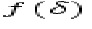represent the transform of the picture at q=1 minus the one at q=0, and designate the spectral image's transform to be f(I), then the square of the error for the i,jth Fourier component is given by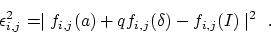(2)
Over an appropriate range of high frequencies in i and j we must solve for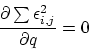(3)
which leads to the expression for q,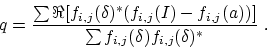(4)

For every image with a spectrum I, we evaluated q and then produced a background corrected version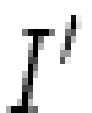from an appropriate linear combination of two template pictures a and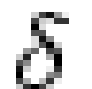,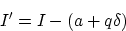(5)
When this subtraction is done, the amplitude of the random checkerboard decreases substantially, and virtually all that remains are other sources of noise.Next: Identification of Bad Pixels Up: Data Reduction Previous: Removal of Electronic Interference

12/15/1998